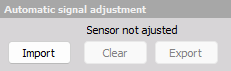# Counter math (Offline)

With the Counter math module it is possible to recalculate counter angle- and frequency outputs offline, during datafile analysis.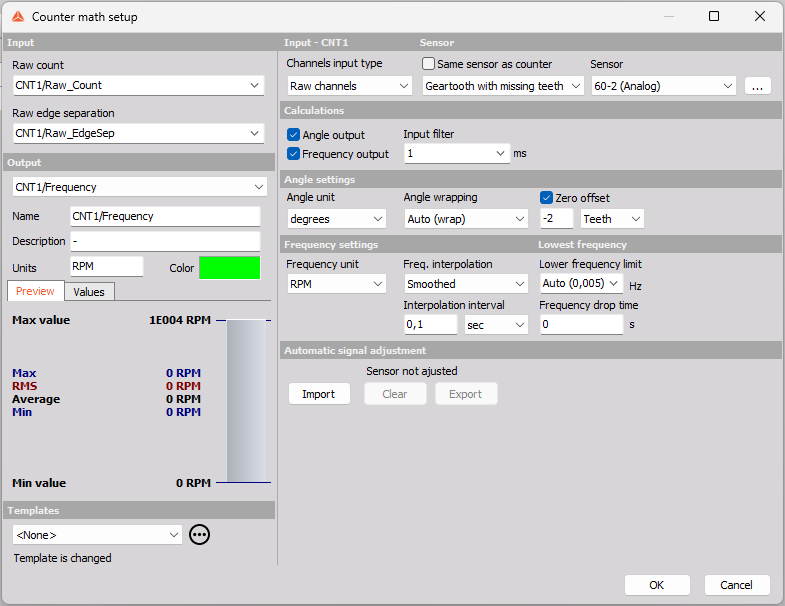This can be useful when you e.g. want to fix a faulty counter setting that wasn’t caught during the measurement, or if you want to tune a setting or two to see if that produces better results.

NOTE: Remember to store the “Raw edge separation” and “Raw count” channels during the measurements, since such data is required to perform the recalculation.

Counter math supports features like frequency smoothing, teeth size adjustment, software filters, and angle wrapping, all described in sections below.

If the results from Counter math turn out to be better for your specific datafile, you can also use the math output as input to other modules that work with counters, such as Order Tracking or Torsional Vibration.

## Input channels

The Counter math module supports both counter channels and raw channels as inputs to process the angle and frequency outputs.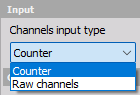### Counter

If you select Counter as Input type, then you must select a Counter channel as input on the left side of the setup page: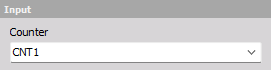### Raw channels

If you select Raw channels then you have to select two channels as input. One for the raw pulse count and one for the raw edge separation: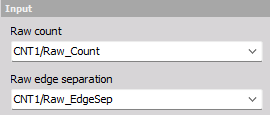Together the raw count channel and the raw edge separation channel can be used like a Counter input to recalculate the angle and frequency outputs with Super Counter precision.

## Sensor

By default the expected sensor type is set equal to the sensor type used in the related Counter channel module, for the Input channel.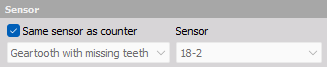### Sensor type

The sensor type can be changed manually by un-checking the “Same sensor as counter” checkbox: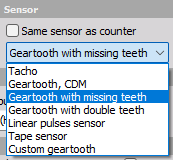In that case the Sensor configuration must also be selected or defined under the three dots […]: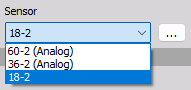## Calculations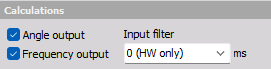Select which results you want. You can check on Angle (phase) data, Frequency (speed) data or both.

### Input filter

On top of the used Counter hardware Input filter you can enable a Counter math software Input filter.

The SW filter can be used to prevent double counts. The filter should be set to react a bit faster than the expected event/pulse rate, and at the same time it should be set a bit slower than the time interval where signal glitches are expected.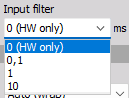If set to 0 then only the Counter HW filter will be used.

## Angle settings

Parameters related to the Angle output channel.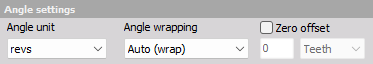### Angle unit

The output angle data can be set to have one of the following units:

• Revs - revolutions and fractions of revolutions
• degrees - 0º - 360º if wrapped
• Counts - number of pulses and fraction of pulses.
• Radians - 0 - 2π, if wrapped.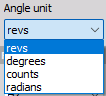### Angle wrapping

The angle data can be set to Wrap back and start from 0 again when a full rotation has appeared. By selecting Unwrap the angle data will continue to accumulate angles over multiple rotations.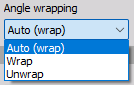### Zero (angle) offset

The Zero angle offset can be shifted either by a number of gear teeth or by a specific angle defined in degrees: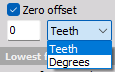## Frequency settings

Parameters related to the Frequency output channel.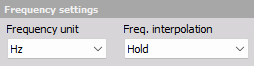### Frequency unit

The output frequency data can be set to have one of the following units:

• RPM - Rounds Per Minute
• Hz - Rounds per second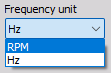### Freq. interpolation

The frequency or speed data values calculated in-between pulses (between raw-count steps) can be handled in multiple ways. The following interpolation types are supported:

• Hold - No interpolation. Instead the speed value is kept until the next raw count step occurs.
• Linear - Linear interpolation between raw count steps.
• Smoothed - Moving averaged speeds with a user-defined averaging time or number of revolutions.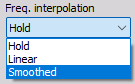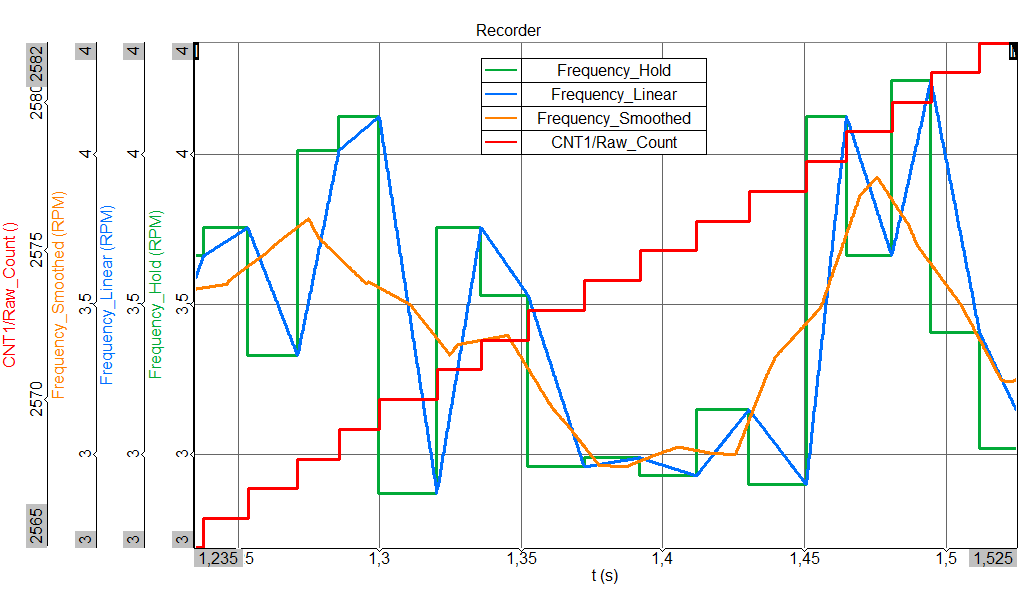In the Picture above the three interpolation types are compared together with the related raw count data.

#### Interpolation interval (Smoothed)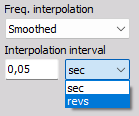When Freq. interpolation is set to Smoothed, then the smoothing strength can be adjusted. The Smoothing interpolation is a linear moving average where the moving average interval is the user-defined Interpolation interval.

The Interpolation interval can have either a fixed time length set in seconds, or a fixed angle length set in number of revolutions.

## Lowest frequency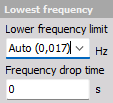If the measured frequency or speed drops below the set Lower frequency limit, then it is assumed to be 0.

If the speed suddenly drops below the Lower frequency limit, then the Counter math frequency output will generate an artificial ramp down towards 0 over the specified Frequency drop time.